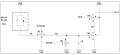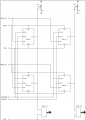# How to approach the design of a pixel circuit using MOSFETs

#### sijafae

Joined Nov 12, 2020
4
Good Day,

I am working on a school project in which we are supposed to decide the component sizes of transistors and capacitors in order for the 4-pixel camera to function properly. I have so far come up with some calculations which I am not very confident is the correct way to approach this problem and I hope I can get some feedback on what I have done wrong so far, and how I should approach the problem.

To begin with, it might be good with some background information as to what I am supposed to do:The first picture shows the pixel circuit. Pins Expose, Erase, and NRE (or Read_n) are digital control signals that we are going to create in Verilog. How these signals, and the other control signals work, are shown in the waveform diagram above. The NRE signal is used to select between 2-pixel circuits in a column to be available at the ADC input pin. Erasing the charge of Cs by using the Erase pin has to occur before Expose can happen because no voltage should be present on Cs before allowing the diode current to be stored in it, otherwise it will seem as if a different lighting strength is present than there actually is. The amount of time of exposing can be anything between 2ms and 30ms, while the diode current is 50pA (very weak lighting) to 750pA (strong lighting). When there is little light, generally the amount of exposure time should be higher while much light should have a lower expose time. It is possible to set the exposure time manually, but the default is that 30ms corresponds to 50pA, while 2ms corresponds to 750pA.

The picture below shows a picture of the 2x2 pixel camera, where Mc1 and Mc2 are active loads each connected to a column of pixel circuits. When a pixel in each column is selected with the NRE signal, the ADC signal will go high the next clock cycle to digitize the values of the outputs of the pixel circuits in parallel.I have attached the calculations I have performed so far, although those notes may be hard to read. I can in case write a short summary of what I have been thinking:
Constraints:
1. 0.36μm < L < 1.08μm
2. 1.08μm < W < 5.04μm
3. 50pA < $I_{pd}$ < 750pA
4. 2ms < t < 30ms
5. 180nm transistor technology
6. $V_{DD}$ = 1.8V
7. $C_c$ = 3pF (parasitic capacitance)
8. $C_s \leq 3pF$
9. f = 1kHz

Firstly, I tried to figure out how each transistor functioned in the circuit:
1. M1: When transistor M1 goes active, it allows the constant current from the photodiode to pass through and be stored in the capacitor, Cs. (Operates in the Active region).
2. M2: When transistor M2 goes active, Cs will be discharged through the transistor to the ground. (Using formula $i = C*\frac{dV}{dt}$ to find the amount of time required to discharge the capacitor and use the MOSFET size to decide current?) (Operates in the active region)
3. M3: This is a buffer stage (source follower). The amount of charge stored in Cs will decide the current through this transistor. When there is no light, no charge will be present in the capacitor, and the current through the transistor will be at its maximum. The current and exposure time combination is at the proper value, the capacitor charge will reach its maximum, which I can decide is the value in which transistor M3 is Off. Thus, when the exposure and the current combination is correct for the lighting, the value the ADC will see is a constant value. (Operates in the active region)
4. M4: Not-read transistor. When the NRE pin goes low, the transistor will become active. In my calculations, I figured that this transistor can not be in the active region, and must operate in the triode region due to the overdrive voltage always being higher than the source-drain voltage.
5. MC: This is the active load transistor connected to each column of pixel circuits. When the circuit is idle, capacitor Cc will be charged by the transistor, due to the overdrive voltage always being one threshold voltage below the source-drain voltage, making the transistor be in the active region. When the capacitor charge has reached Vdd - |Vtp|, the transistor will turn off due to the overdrive voltage reaching 0V.

Now that I have (hopefully) figured out the transistor functionalities, I begin looking for other constraints that will help me decide the component values. I decide to first figure out the lower boundary of the capacitor as well as finding the appropriate formula to later calculate the value. Since the current going through the photodiode is constant, the capacitor charging formula can be simplified to: $C_s = \frac{I_{pd}*t}{V_{cs}}$.
Figuring out the upper boundary of $V_{cs}$ will give the lower boundary of $C_s$. To figure out the upper boundary of $V_{cs}$, I can look at a few conditions for M3 and MC that must be met in order for them to always be conducting current:
1. $V_{DD} - V_{out} = V_{SG\_C} > |V_{tp}|$
2. $V_{S\_4} - V_{cs} = V_{SG\_3} > |V_{tp}|$
By setting $V_{cs} = V_{out}$, transistor M3 will still be conducting, but no current will be present due to M4 having $V_{SD} = 0$. Using this, and the conditions stated above, it can be shown that $V_{cs} < V_{DD} - 2*|V_{tp}|$. Placing this condition in for $V_{cs}$ in the capacitor formula, it can be shown that: $1.67pF < C_s \leq 3pF$.
Now that the range of $C_s$ is figured out, I decided to solve the size ratio between the transistors M3 and M4. Here, I looked at the transistors when the maximum current is present, that is when $V_{cs} = 0$. Knowing that transistor M4 is operating in the triode region, I decided that having $V_{SD\_4}|_{max} = |V_{tp}|$, that way I can approximate the current to be using the formula: $I_{s4} = \mu_p*C_{ox}*(\frac{W}{L}*k_4)*V_{eff\_4}*V_{SD\_4}$. By a few calculations (shown in the handwritten notes, however I calculated $V_{eff\_4}$ incorrectly, where it is supposed to be $V_{eff\_4} = V_{DD} - |V_{tp}| = 3*|V_{tp}|$, so the ratio should be $K_3 = \frac{3K_4}{2}$), the size relationship can be decided. Here I used the following values and relationships to solve for the size ratio:
1. $I_{S\_3} = I_{S\_4} \\ \frac{\mu_p*C_{ox}}{2}*(\frac{W}{L}*K_3)*V_{eff\_3}^2 = \mu_p*C_{ox}*(\frac{W}{L}*K_4)*V_{eff\_4}*V_{SD\_4}$
2. $V_{eff\_3} = |V_{tp}|$
3. $V_{eff\_4} = 3*|V_{tp}|$
4. $V_{SD\_4} = |V_{tp}|$
Using these formulas for the equal currents and the values for the transistor voltages, it can be shown that the size relationship between transistor M3 and M4 is: $k_3 = \frac{3*k_4}{2}$.

This is as far as I have gotten so far. I am unsure about how I should solve for the discharge of $C_c$, but maybe I can use the formula $i = C*\frac{dV}{dt}$ and then set the current going through transistors M3 and M4 equal to the sum of the capacitor discharge current and the current that will be produced in transistor MC when the voltage over the capacitor starts dropping? As I can see, the source-gate voltage of MC will increase as the capacitor is discharged, while the source-drain voltage will always be high enough to drive the transistor in the active region. Then, since the transistor only varies by the source gate voltage (exponential), the discharge current of the parasitic capacitor $C_c$ and the transistor current of MC are both exponentially changing currents. In the end, these currents will sum up to the current of $I_{S\_3}$. That is, $I_{S\_3} = I_c+I_{S\_c}$. Using this relationship, the lower boundary that the output voltage will reach before the voltage is stabilizing due to no more current being discharged from the parasitic capacitor, or rather, the charging current from the transistor outweighs the discharging of the capacitor.

I have attached my handwritten notes to this post in order to give a more thorough overview of what I have done (sorry for horrible handwriting).

Thank you for taking the time to read this. I hope someone could please help me get on the right path to solving this problem. Any help, tips, or suggestions would be very useful and I would greatly appreciate it.

Have a great day,
Sindre

#### Attachments

• 3.2 MB Views: 4